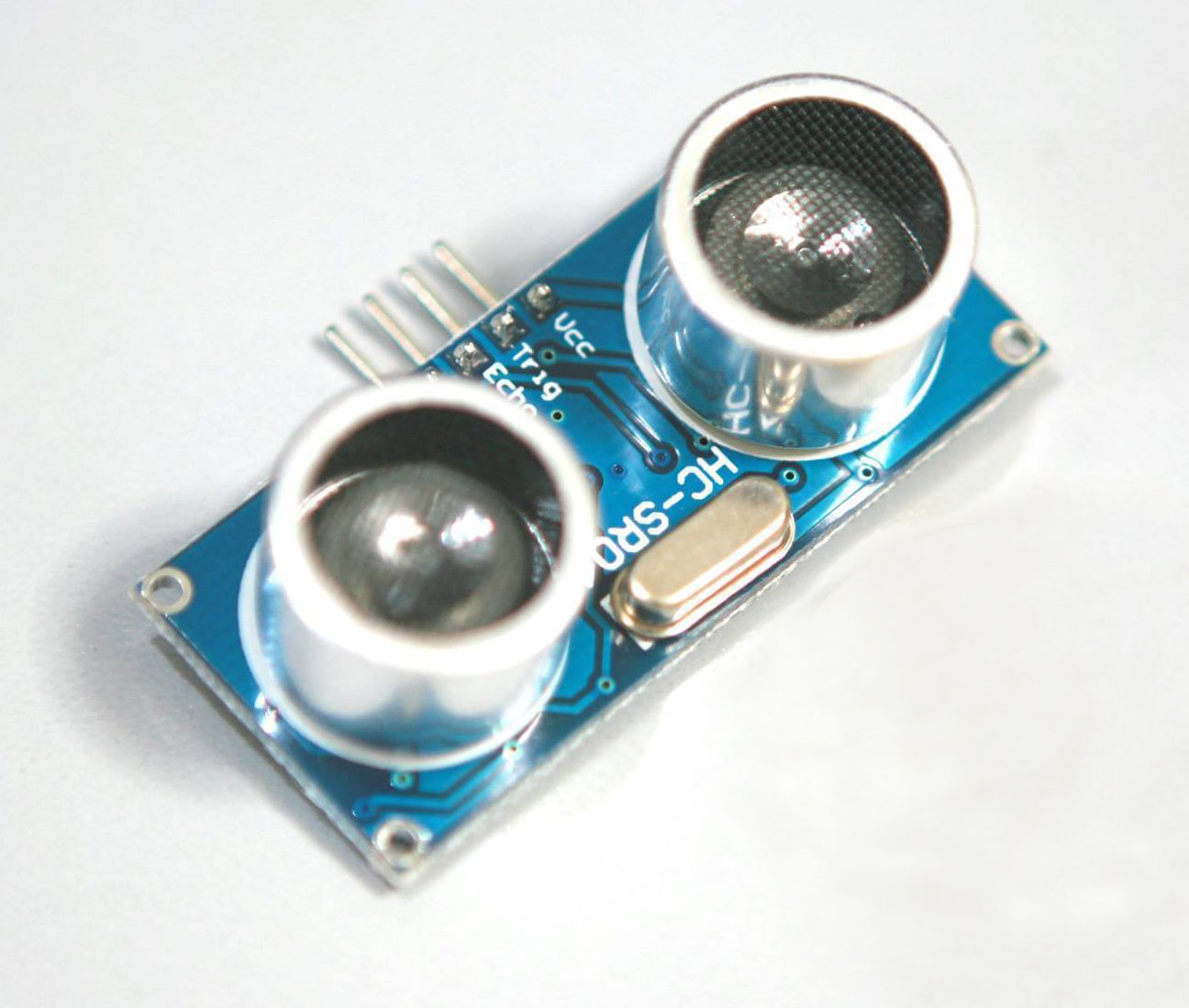# HC-SR04 and Arduino Interfacing Tutorial

An ultrasonic sensor like the HC-SR04 finds a lot of applications because of its low cost and ease of use.

### Introduction

Ultrasonic literally means "sound beyond what humans hear" which is the signal emitted by the module. By measuring when the signal is emitted and when it is sent back to the receiver, we can measure the distance between the ultrasonic sensor and the body that reflects the signal. In this tutorial, we will use the HC-SR04 with an Arduino to measure the distance from an obstacle.

### The HC-SR04 Module

The HC-SR04 module has four pins (Vcc, Trigger, Echo, Gnd) as shown:When the trigger pin is held high, the module begins emitting ultrasonic signal until the pin is pulled low. If the signal encounters an obstacle, it will return back to the module. As a result, the "echo" of the signal is used to measure the distance: the duration between the sending of the signal and the return of the signal can be read on the echo pin as a pulse.

The distance in centimeters between the HC-SR04 and the obstacle can then be calculated using

where 0.034 is derived from the speed of sound: 340 m/s

### Arduino Sketch

Here is an Arduino sketch that measures the distance of an obstacle and prints it on the serial monitor:

```// Trigger Pin -> Arduino Pin 9
// Echo Pin -> Arduino Pin 10

const int trigger = 9;
const int echo = 10;

// defines variables
long _time;
int distance;

void setup() {
pinMode(trigger, OUTPUT);
pinMode(echo, INPUT);
Serial.begin(9600);
}

void loop() {
// This sequence is required to clear the trigger pin
digitalWrite(trigger, LOW);
delayMicroseconds(2);

// Send a 10 micro second pulse
digitalWrite(trigger, HIGH);
delayMicroseconds(10);
digitalWrite(trigger, LOW);

// Reads the echo pin for the duration of the signal travel
duration = pulseIn(echo, HIGH);

// distance = duration * speed of sound / 2
distance= duration*0.034/2;

// Prints the distance on the Serial Monitor
Serial.print("Distance: ");
Serial.print(distance);
Serial.print(“ cm”);
}
```

This ultrasonic sensor module can also be used to measure the presence of an obstacle in front of the module. See my hexapod walker robot project where I used the HC-SR04 to detect an obstacle in front of a robot.